• +91 9971497814
• info@interviewmaterial.com

# Arithmetic Progressions Ex-5.4 Interview Questions Answers

### Related Subjects

Question 1 : Which term of the AP: 121, 117, 113, . . ., isits first negative term? [Hint: Find n for an < 0]

Given the AP series is 121, 117, 113, . . .,

Thus, first term, a = 121

Common difference, d = 117-121= -4

By the nth term formula,

an = a+(n −1)d

Therefore,

an = 121+(n−1)(-4)

= 121-4n+4

=125-4n

To find the first negative term of theseries, a< 0

Therefore,

125-4n < 0

125 < 4n

n>125/4

n>31.25

Therefore, the first negative term of the series is 32nd term.

Question 2 : The sum of the third term and the seventh term of an AP is 6 and their product is 8. Find the sum of first sixteen terms of the AP?

Answer 2 :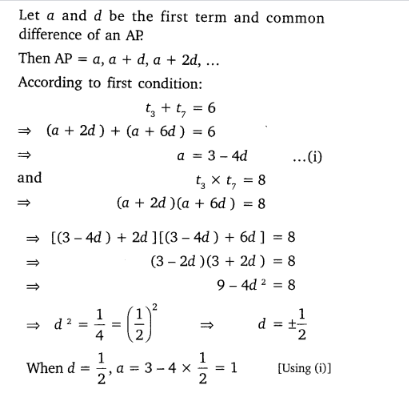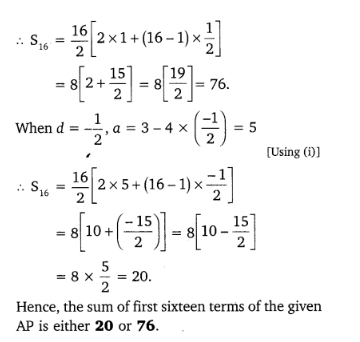Question 3 : A ladder has rungs 25 cm apart. (see Fig.5.7). The rungs decrease uniformly in length from 45 cm at the bottom to 25 cmat the top. If the top and the bottom rungs are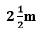apart, what is the length of the woodrequired for the rungs?

Answer 3 :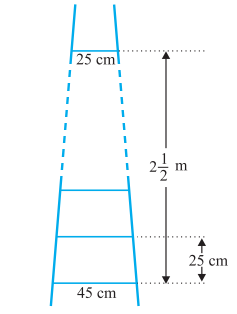Solution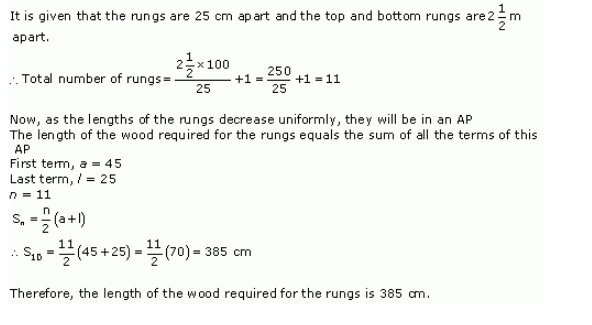Question 4 : The houses of a row are numbered consecutivelyfrom 1 to 49. Show that there is a value of x such that the sum of the numbersof the houses preceding the house numbered x is equal to the sum of the numbersof the houses following it. Find this value of x.

Answer 4 :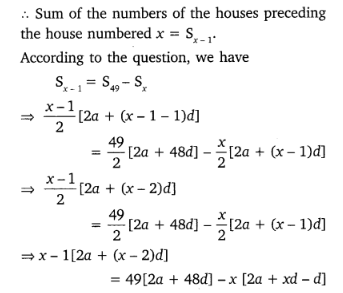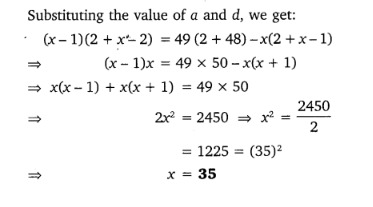Question 5 : A small terrace at a football ground comprises of 15 steps each of which is 50 m long and built of solid concrete.

Each step has a rise of 1 4 m and a tread of 1 2 m. (see Fig. 5.8). Calculate the total volume of concrete required to build the terrace.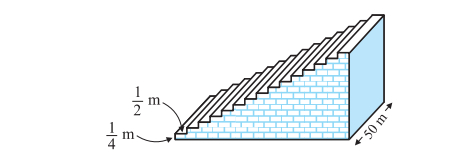Solution

As we can see from the given figure, the firststep is ½ m wide, 2nd step is 1m wide and 3rd stepis 3/2m wide. Thus we can understand that the width of step by ½ m each timewhen height is ¼ m. And also, given length of the steps is 50m all the time.So, the width of steps forms a series AP in such a way that;

½ , 1, 3/2, 2, ……..

Volume of steps = Volume of Cuboid

Now,

Volume of concrete required to build the firststep = ¼ ×1/2 ×50 = 25/4

Volume of concrete required to build thesecond step =¼ ×1/×50 = 25/2

Volume of concrete required to build thesecond step = ¼ ×3/2 ×50 = 75/2

Now, we can see the volumes of concreterequired to build the steps, are in AP series;

25/4 , 25/2 , 75/2 …..

Thus, applying the AP series concept,

First term, a = 25/4

Common difference, d = 25/2 – 25/4 = 25/4

As we know, the sum of n terms is;

Sn = n/2[2a+(n-1)d] =15/2(2×(25/4 )+(15/2 -1)25/4)

Upon solving, we get,

Sn = 15/2 (100)

Sn750

Hence, the total volume of concrete requiredto build the terrace is 750 m3.

Todays Deals### Arithmetic Progressions Ex-5.4 Contributorskrishan

Name:
Email:

# Latest News# 9000 interview questions in different categories